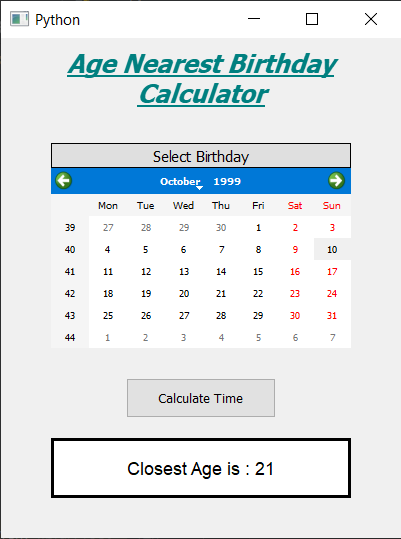Related Articles

# PyQt5 – Age Nearest Birthday Calculator

• Last Updated : 21 Jun, 2020

In this article we will see how we can create a age nearest birthday calculator. Age nearest calculator is the calculator which is used to get the age nearest to the birth date. Below is how the age nearest calculator looks likeConcept : We can get the age nearest birthday by getting the difference between the birth date and the present date with the help of python datetime objects will keep count of leap years and we can get the number of days between the dates. Then these days can be divided with the 365.32422 to get the years, then the round value of years will be the age nearest birthday

GUI Implementation Steps :
1. Create a heading label that display the calculator name
2. Create label to show user to select the birth date
3. Create a QCalendarWidget object for user to select the birth date
4. Create a push button to calculate the nearest age
5. Create a label to show the calculated age

Back-End Implementation :
1. Make the calendar future date block i.e set current date as maximum date
2. Add action to the push button
3. Inside the push button action get the date from the calendar and the present date
4. Get the day, month and year from both the dates
5. Create a datetime.date object for both the dates
6. Get the difference from both the dates and get the days
7. Divide the days with 365.2422(due to leap year) to get total years
8. Get the round value of the years and show this value through label

Below is the implementation

 `# importing libraries``from` `PyQt5.QtWidgets ``import` `*` `from` `PyQt5 ``import` `QtCore, QtGui``from` `PyQt5.QtGui ``import` `*` `from` `PyQt5.QtCore ``import` `*` `import` `datetime``import` `sys`` ` ` ` `class` `Window(QMainWindow):`` ` `    ``def` `__init__(``self``):``        ``super``().__init__()`` ` `        ``# setting title``        ``self``.setWindowTitle(``"Python "``)`` ` `        ``# width of window``        ``self``.w_width ``=` `400`` ` `        ``# height of window``        ``self``.w_height ``=` `500`` ` `        ``# setting geometry``        ``self``.setGeometry(``100``, ``100``, ``self``.w_width, ``self``.w_height)`` ` `        ``# calling method``        ``self``.UiComponents()`` ` `        ``# showing all the widgets``        ``self``.show()`` ` `    ``# method for components``    ``def` `UiComponents(``self``):`` ` `        ``# creating head label``        ``head ``=` `QLabel(``"Age Nearest Birthday Calculator"``, ``self``)`` ` `        ``head.setWordWrap(``True``)`` ` `        ``# setting geometry to the head``        ``head.setGeometry(``0``, ``10``, ``400``, ``60``)`` ` `        ``# font``        ``font ``=` `QFont(``'Times'``, ``15``)``        ``font.setBold(``True``)``        ``font.setItalic(``True``)``        ``font.setUnderline(``True``)`` ` `        ``# setting font to the head``        ``head.setFont(font)`` ` `        ``# setting alignment of the head``        ``head.setAlignment(Qt.AlignCenter)`` ` `        ``# setting color effect to the head``        ``color ``=` `QGraphicsColorizeEffect(``self``)``        ``color.setColor(Qt.darkCyan)``        ``head.setGraphicsEffect(color)`` ` `        ``# creating a label``        ``b_label ``=` `QLabel(``"Select Birthday"``, ``self``)`` ` `        ``# setting properties  label``        ``b_label.setAlignment(Qt.AlignCenter)``        ``b_label.setGeometry(``50``, ``105``, ``300``, ``25``)``        ``b_label.setStyleSheet(``"QLabel"``                              ``"{"``                              ``"border : 1px solid black;"``                              ``"background : rgba(70, 70, 70, 25);"``                              ``"}"``)``        ``b_label.setFont(QFont(``'Times'``, ``9``))`` ` `        ``# creating a calendar widget to select the date``        ``self``.calendar ``=` `QCalendarWidget(``self``)`` ` `        ``# setting geometry of the calendar``        ``self``.calendar.setGeometry(``50``, ``130``, ``300``, ``180``)`` ` `        ``# setting font to the calendar``        ``self``.calendar.setFont(QFont(``'Times'``, ``6``))`` ` `        ``# getting current date``        ``date ``=` `QDate.currentDate()`` ` `        ``# blocking future dates``        ``self``.calendar.setMaximumDate(date)`` ` ` ` ` ` ` ` `        ``# creating a push button``        ``calculate ``=` `QPushButton(``"Calculate Time"``, ``self``)`` ` `        ``# setting geometry to the push button``        ``calculate.setGeometry(``125``, ``340``, ``150``, ``40``)`` ` `        ``# adding action to the calculate button``        ``calculate.clicked.connect(``self``.calculate_action)`` ` `        ``# creating a label to show percentile``        ``self``.result ``=` `QLabel(``self``)`` ` `        ``# setting properties to result label``        ``self``.result.setAlignment(Qt.AlignCenter)``        ``self``.result.setGeometry(``50``, ``400``, ``300``, ``60``)``        ``self``.result.setWordWrap(``True``)``        ``self``.result.setStyleSheet(``"QLabel"``                                  ``"{"``                                  ``"border : 3px solid black;"``                                  ``"background : white;"``                                  ``"}"``)``        ``self``.result.setFont(QFont(``'Arial'``, ``11``))`` ` ` ` `    ``def` `calculate_action(``self``):`` ` `        ``# getting birth date day``        ``birth ``=` `self``.calendar.selectedDate()`` ` `        ``# getting year and month day of birth day``        ``birth_year ``=` `birth.year()``        ``birth_month ``=` `birth.month()``        ``birth_day ``=` `birth.day()`` ` `        ``# getting today date``        ``current ``=` `QDate.currentDate()`` ` `        ``# getting year and month day of current day``        ``current_year ``=` `current.year()``        ``current_month ``=` `current.month()``        ``current_day ``=` `current.day()`` ` `        ``# coverting  dates into date object``        ``birth_date ``=` `datetime.date(birth_year, birth_month, birth_day)``        ``current_date ``=` `datetime.date(current_year, current_month, current_day)`` ` `        ``# getting difference in both the dates``        ``difference ``=` `current_date ``-` `birth_date`` ` `        ``# getting days from the difference``        ``difference ``=` `difference.days`` ` `        ``# getting years from the difference``        ``years ``=` `difference ``/` `365.2422`` ` `        ``# getting round value of years``        ``years ``=` `round``(years)`` ` `        ``# setting this value with the help of label``        ``self``.result.setText(``"Closest Age is : "` `+` `str``(years))`` ` ` ` ` ` `# create pyqt5 app``App ``=` `QApplication(sys.argv)`` ` `# create the instance of our Window``window ``=` `Window()`` ` `# start the app``sys.exit(App.``exec``())`

Output :

Attention geek! Strengthen your foundations with the Python Programming Foundation Course and learn the basics.

To begin with, your interview preparations Enhance your Data Structures concepts with the Python DS Course. And to begin with your Machine Learning Journey, join the Machine Learning – Basic Level Course

My Personal Notes arrow_drop_up